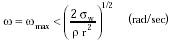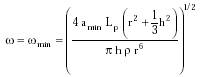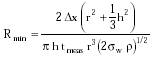Nanomedicine, Volume I: Basic Capabilities

Robert A. Freitas Jr., Nanomedicine, Volume I: Basic Capabilities, Landes Bioscience, Georgetown, TX, 1999

4.3.3.4 Pivoted Gyroscopic Accelerometers

Consider a cylindrical gyroscope spinning at an angular velocity w (radians/sec), with radius r and thickness h, whose center of gravity lies a distance Lp from a frictionless pivot point along the rotational axis. The pivot point defines the center of a spherical sensor grid of radius R (r2 + 4h2)1/2 having distinguishable grid elements located a distance Dx ~ 1 nm apart. Under uniform acceleration a, the gyroscope precesses around its spin axis at an angular velocity wp = 2 a Lp / w r2 = Dx / R tmeas, where tmeas is measurement duration. Hence the minimum detectable acceleration is{Eqn. 4.16}

by comparing precessional motion against the grid.

In choosing an w with which to measure amin, there is an upper and a lower limit. The upper bound is the bursting strength condition{Eqn. 4.17}

where sw is a safe working stress = 1010 N/m2 (~0.2 tensile strength) for diamond10 and r is the density of the gyroscope material (~3510 kg/m3 for diamond). The lower bound is the minimum spin angular velocity below which the pivoted gyroscope cannot spin stably about the vertical axis and begins to wobble,448 thus ruining the measurement. The lower bound is given by{Eqn. 4.18}

If wmax / wmin > 1, then nonwobbling spin velocities are available below the bursting speed of the gyroscope cylinder. Since this ratio scales with R, there is a minimum sensor size Rmin below which no useful spin velocities are available. Combining Eqns. 4.16 and 4.18 and solving the quadratic in w gives{Eqn. 4.19}

For tmeas = 10-3 sec, Rmin >~ 69 microns (optimum r = 48 microns, h = 25 microns); for tmeas = 1 sec, Rmin >~ 6.9 microns (optimum r = 4.8 microns, h = 2.5 microns). Thus it appears that pivoted gyroscopic accelerometers likely will be employed only in devices >> 10 microns in size (but see Section 4.3.4.1). Microscale gyroscopes implemented in silicon have been discussed by Greiff 1383 and others.447

Last updated on 17 February 2003